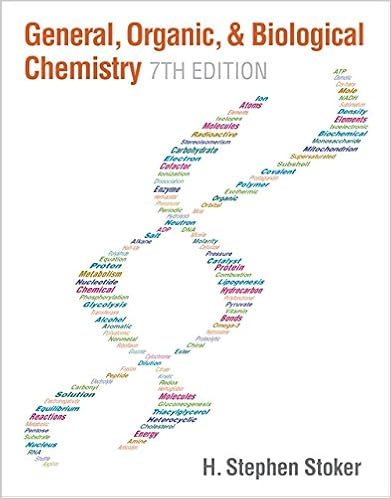# Types of oxidation reduction reactions chlorine

• Notes
• 190

This preview shows page 78 - 89 out of 190 pages.

##### We have textbook solutions for you!
The document you are viewing contains questions related to this textbook.The document you are viewing contains questions related to this textbook.
Chapter 15 / Exercise 15-83
General, Organic, & Biological Chemistry
StokerExpert Verified
Types of Oxidation-Reduction Reactions Chlorine Chemistry 0 +1 -1 78
##### We have textbook solutions for you!
The document you are viewing contains questions related to this textbook.The document you are viewing contains questions related to this textbook.
Chapter 15 / Exercise 15-83
General, Organic, & Biological Chemistry
StokerExpert Verified
Ca2++ CO32-CaCO3NH3+ H+NH4+Zn + 2HCl ZnCl2+ H2Ca + F2CaF2Precipitation Acid-Base Redox (H2Displacement) Redox (Combination) Classify the following reactions. 79
Oxidation-Reduction (Redox) Reactions Oxidationis the lossof electrons. The reducing agentloses electrons and is oxidized.Reductionis the gainof electrons. The oxidizing agentgains electrons and is reduced.A redox reactioninvolves electron transferOxidation and reduction occur together.
Figure 4.12 The redox process in compound formation.
1. For an atom in its elemental form (Na, O2, Cl2, etc.): O.N. = 0 2. For a monoatomic ion: O.N. = ion charge 3. The sum of O.N. values for the atoms in a compound equals zero. The sum of O.N. values for the atoms in a polyatomic ion equals the ion’s charge.General rules Rules for specific atoms or periodic table groups 1. For Group 1A(1): O.N. = +1 in all compounds 2. For Group 2A(2): O.N. = +2 in all compounds 3. For hydrogen: O.N. = +1 in combination with nonmetals 4. For fluorine: O.N. = -1 in combination with metals and boron 6. For Group 7A(17): O.N. = -1 in combination with metals, nonmetals (except O), and other halogens lower in the group 5. For oxygen: O.N. = -1 in peroxides O.N. = -2 in all other compounds(except with F) Table 4.3 Rules for Assigning an Oxidation Number (O.N.)
Sample Problem 4.9 Determining the Oxidation Number of Each Element in a Compound (or Ion) PROBLEM: Determine the oxidation number (O.N.) of each element in these species: (a) zinc chloride(b) sulfur trioxide(c) nitric acidPLAN: SOLUTION: The O.N.s of the ions in a polyatomic ion add up to the charge of the ion and the O.N.s of the ions in the compound add up to zero. (a) ZnCl2. The O.N. for zinc is +2 and that for chloride is -1.(b) SO3. Each oxygen is an oxide with an O.N. of -2. The O.N. of sulfur must therefore be +6.(c) HNO3. H has an O.N. of +1 and each oxygen is -2. The N must therefore have an O.N. of +5.
Sample Problem 4.10 Identifying Redox Reactions PROBLEM: Use oxidation numbers to decide whether each of the following is a redox reaction or not. (a)CaO (s) + CO2 (g) CaCO3 (s)(b)4 KNO3 (s) 2 K2O(s) + 2 N2(g)+ 5 O2(g) (c)NaHSO4 (aq) + NaOH (aq) Na2SO4 (aq) + H2O (l) PLAN: Use Table 4.3 to assign an O.N. to each atom. A change in O.N. for any atom indicates electron transfer. SOLUTION: (a)CaO(s) + CO2(g) CaCO3(s) This is not a redox reaction, since no species change O.N. +2 -2 -2 +2 +4 -2 +4
Sample Problem 4.10 (b)4 KNO3 (s) 2 K2O(s) + 2 N2(g)+ 5 O2(g) This is a redox reaction. N changes O.N. from +5 to 0 and is reduced. O changes O.N. from -2 to 0 and is oxidized. +5 -2 -2 0 +1 +1 0
Sample Problem 4.10 This is not a redox reaction since no species change O.N. (c)NaHSO4 (aq) + NaOH (aq) Na2SO4 (aq) + H2O (l) +6 -2 -2 +1 +1 +1 +1 +1 +6 -2 +1 -2
Figure 4.13 A summary of terminology for redox reactions.
Sample Problem 4.11 Identifying Oxidizing and Reducing AgentsPROBLEM:Identify the oxidizing agent and reducing agent in each of the following reactions: (a)2Al (s) + 3H2SO4 (aq) Al2(SO4)3 (aq) + 3H2(g)(b)PbO (s) + CO (g) Pb (s) + CO2 (g) (c)2H2 (g) + O2 (g) 2H2O (g) PLAN:
•••